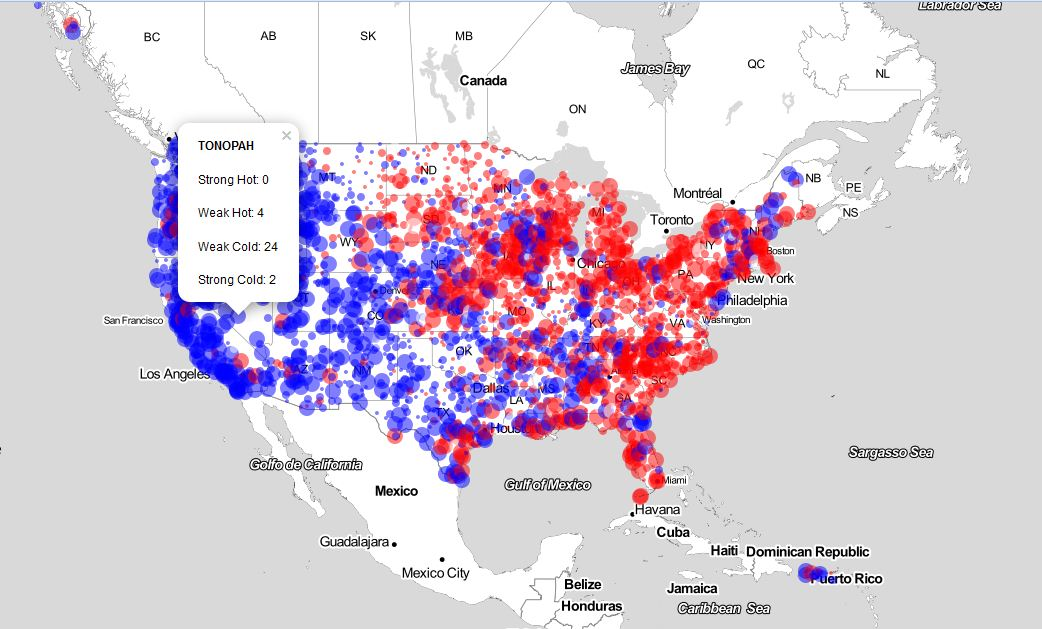# Climatic Change At A Glance

Mmm. Lost a planet, Master Obi-Wan has. How embarrassing (Yoda, Attack Of The Clones)

Some time ago I published this post in KDnuggets in which I analyze historical temperatures to show how we are gradually heading toward a warmer planet. Simple data science to obtain a simple (and increasingly accepted) conclusion: the global warming is real. Despite I was criticized I still believe what I said then: you don’t have to be a climatologist to empirically confirm global warming.

This experiment is another example of that. It is still simpler than that since it is only based on visual perception but I think is also quite conclusive. In this case, I represent U.S. temperature outliers from 1964 to 2013; a map per year. Dataset contains station ID, name, min/max temperature, as well as degree coordinates of the recorded weather. Original weather data collected from NOAA and anomalies analysis by Enigma. You can download data here.

Anomalies are divided into four categories: Strong Hot, Weak Hot, Weak Cold and Strong Cold. For each station, I represent difference between number of Cold and Hot anomalies (independently of the strength) so Blue bubbles represent stations where total number of Cold anomalies during the year is greater that total number of Hot ones and Red ones represent the opposite. Size of bubbles is also proportional to this indicator. As an example, following you can see the map of year 1975:It seems 1975 was hot in the right a cold on the left side. Concretely, in TONOPAH Station (Nevada) were registered 30 anomalies and most of them (26) where due to cold temperatures. This is why bubble is blue. This GIF shows the evolution of all these maps from 1964 to 2013:Maybe it is just my personal feeling but don’t you see how red bubbles are gradually winning to blue ones? Maybe I am a demagogue.

This code generates a dynamic map by year in html format:

```library(data.table)
library(stringr)
library(leaflet)
library(RColorBrewer)
library(classInt)
library(dplyr)
library(htmlwidgets)
anomalies %>%
mutate(year=substr(date_str, 1, 4)) %>%
group_by(year, longitude, latitude, id, station_name) %>%
summarise(
Strong_Hot=sum(str_count(type,"Strong Hot")),
Weak_Hot=sum(str_count(type,"Weak Hot")),
Weak_Cold=sum(str_count(type,"Weak Cold")),
Strong_Cold=sum(str_count(type,"Strong Cold")),
total=n()) %>%
mutate(score=sign(-Strong_Hot-Weak_Hot+Weak_Cold+Strong_Cold)) %>%
mutate(color=ifelse(score==1, "Blue",ifelse(score==0, "White", "Red"))) -> anomalies2
for (i in unique(anomalies2\$year))
{
anomalies2 %>%
filter(year==i) %>%
leaflet() %>%
fitBounds(-124, 34, -62, 40) %>%
lat = ~latitude,
radius = ~ifelse(total < 20, 2, ifelse(total < 27, 4, 8)),
color= ~color,
stroke=FALSE,
fillOpacity = 0.5,
popup = ~paste(sep = "
", paste0("<b>", station_name, "</b>"),
paste0("Strong Hot: ", Strong_Hot),
paste0("Weak Hot: ", Weak_Hot),
paste0("Weak Cold: ", Weak_Cold),
paste0("Strong Cold: ", Strong_Cold))) -> m
saveWidget(m, file=paste0("m", i, ".html"))
}
```

# Simple Data Science Of Global Warming In KDnuggets

Would love to get a post from you for KDnuggets (Gregory Piatetsky, KDnuggets President)Some days ago, Gregory Piatetsky invited me to write a post for KDnuggets. I couldn’t say no. He suggested to me some topics and I decided to experiment around climate change to demonstrate how easy is to see some evidences of this alarming menace. You can read the post here.

This is the code I wrote to do this experiment:

```require(sqldf)
require(ggplot2)
require(ggthemes)
setwd("YOUR WORKING DIRECTORY HERE") #This line doen's work until you type a valid path
files=list.files(pattern = "TG_STAID")
results=data.frame(staid=character(0), trnd=numeric(0), nobs=numeric(0))
#Loop to calculate linear trends
for (i in 1:length(files))
{
table=table[table\$Q_TG==0,]
table\$date=as.Date(as.character(table\$DATE), "%Y%m%d")
results=rbind(data.frame(staid=files[i], trnd=lm.fit (x = matrix(table\$date), y = table\$TG)\$coefficients, nobs=nrow(table)), results)
}
write.csv(results, file="results.csv", row.names = FALSE)#Save your work
#Remove outliers
results=results[!results\$trnd %in% boxplot.stats(results\$trnd, coef = 4)\$out,]
#Histogram
hist(x=results\$trnd, breaks = 50,
col = "orange",
border = "pink",
freq=TRUE,
xlim=c(-0.03, 0.03),
ylim=c(0, 300),
xlab="Historical trend of daily mean temperatures",
ylab="Number of stations",
main="Evolution of temperatures in Europe and the Mediterranean",
sub="Source: European Climate Assessment & Dataset project")
results\$staid2=as.numeric(gsub("[^0-9]","",results\$staid)) #To join results with geographical coordinates
#Right tail of the distribution
hotpoints=sqldf("SELECT a.staid, a.staid2, a.trnd, a.nobs, b.staname, b.lat, b.lon
FROM results a INNER JOIN staids b ON (a.staid2=b.staid) WHERE TRND >= 0.02")
#Convert degrees:minutes:seconds to decimal coordinates
hotpoints=within(hotpoints, {
dms=do.call(rbind, strsplit(as.character(LAT), ":"))
lat=sign(as.numeric(dms[,1]))*(abs(as.numeric(dms[,1]))+(as.numeric(dms[,2]) + as.numeric(dms[,3])/60)/60);rm(dms)
})
hotpoints=within(hotpoints, {
dms=do.call(rbind, strsplit(as.character(LON), ":"))
lon=sign(as.numeric(dms[,1]))*(abs(as.numeric(dms[,1]))+(as.numeric(dms[,2]) + as.numeric(dms[,3])/60)/60);rm(dms)
})
#To make readable tha name of the station in the map
hotpoints\$staname=gsub("^\\s+|\\s+\$", "", hotpoints\$STANAME)
#To prepare coordinates to gvis function
hotpoints\$LatLong=with(hotpoints, paste(lat, lon, sep=":"))
#The amazing gvisMap function of googleVis package
hotpointsMap=gvisMap(hotpoints, "LatLong" , "STANAME",
options=list(showTip=TRUE,
showLine=TRUE,
enableScrollWheel=TRUE,
mapType='terrain',
useMapTypeControl=TRUE))
plot(hotpointsMap)
#The plot one of this hot stations